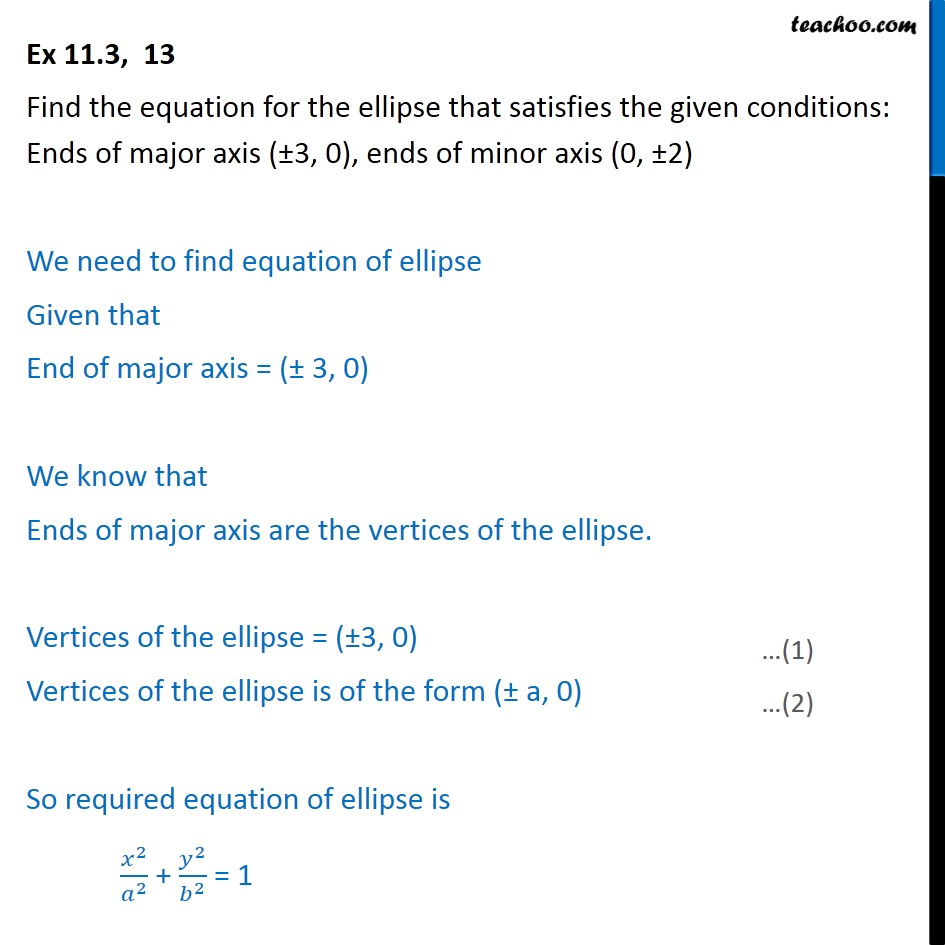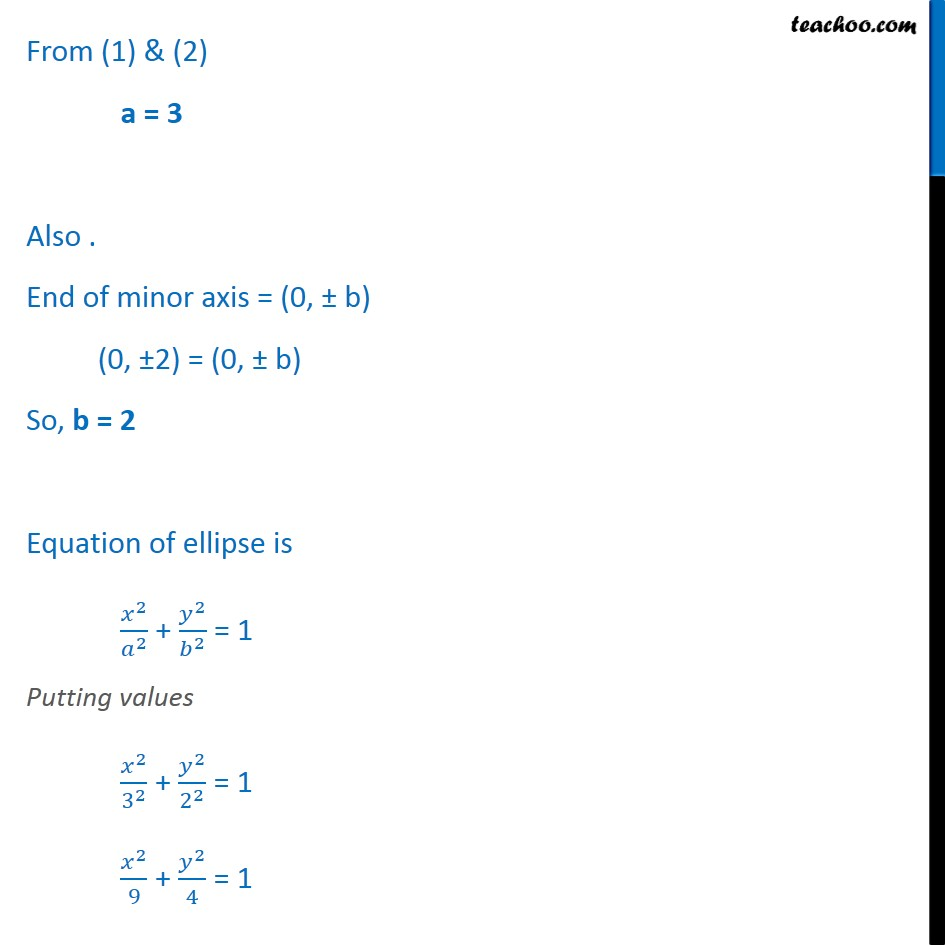Ellipse - Defination

Chapter 10 Class 11 Conic Sections
Concept wiseLearn in your speed, with individual attention - Teachoo Maths 1-on-1 Class

### Transcript

Ex 10.3, 13 Find the equation for the ellipse that satisfies the given conditions: Ends of major axis ( 3, 0), ends of minor axis (0, 2) We need to find equation of ellipse Given that End of major axis = ( 3, 0) We know that Ends of major axis are the vertices of the ellipse. Vertices of the ellipse = ( 3, 0) Vertices of the ellipse is of the form ( a, 0) So required equation of ellipse is 2 2 + 2 2 = 1 From (1) & (2) a = 3 Also . End of minor axis = (0, b) (0, 2) = (0, b) So, b = 2 Equation of ellipse is 2 2 + 2 2 = 1 Putting values 2 3 2 + 2 2 2 = 1 2 9 + 2 4 = 1